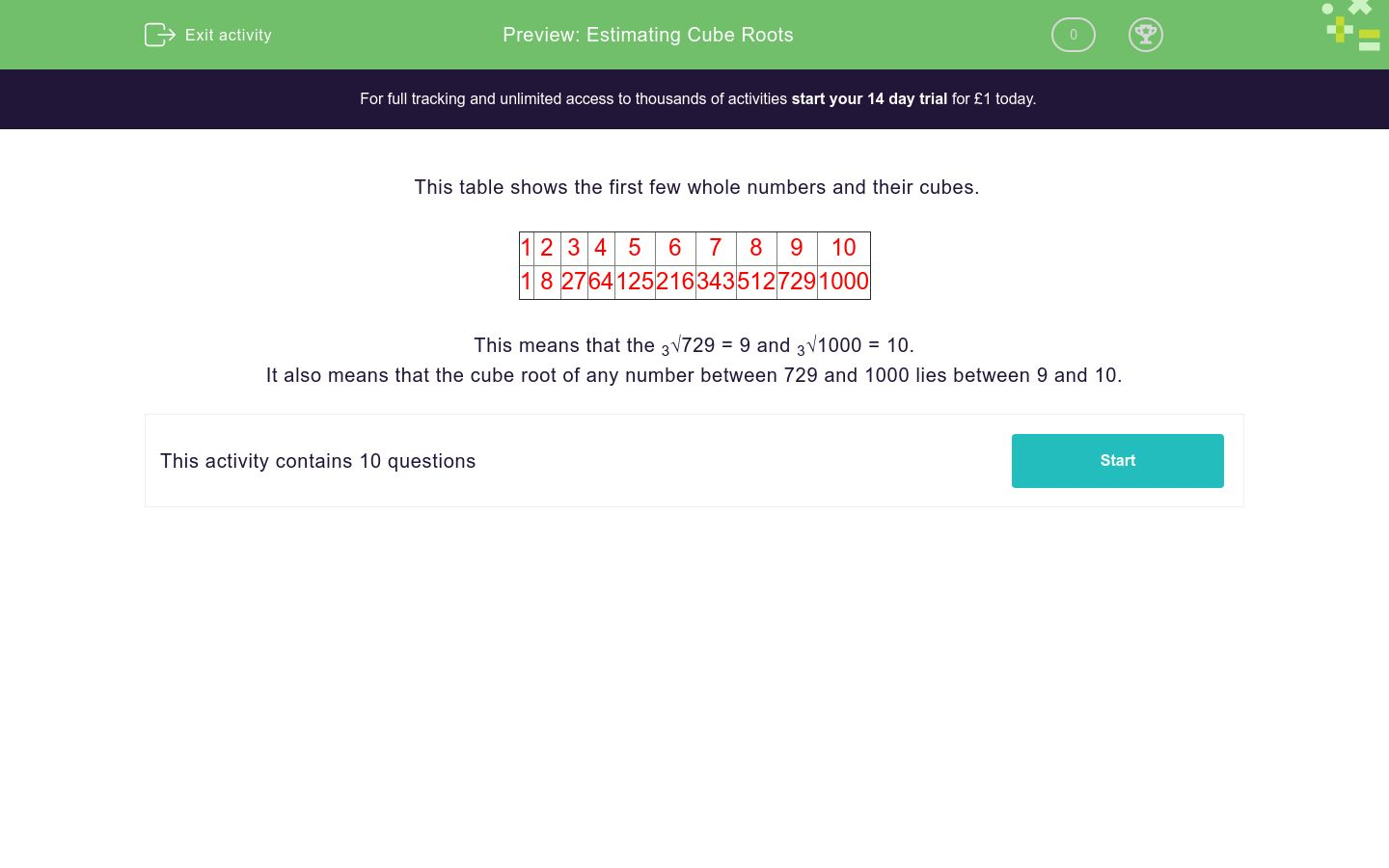# Estimating Cube Roots

In this worksheet, students estimate cube roots.Key stage:  KS 3

Curriculum topic:   Number

Curriculum subtopic:   Understand Integer Powers/Real Roots

Difficulty level:### QUESTION 1 of 10

This table shows the first few whole numbers and their cubes.

 1 2 3 4 5 6 7 8 9 10 1 8 27 64 125 216 343 512 729 1000

This means that the 3√729 = 9 and 3√1000 = 10.

It also means that the cube root of any number between 729 and 1000 lies between 9 and 10.

Between which two numbers does the following cube root lie?

3√10

7 and 8

2 and 3

3 and 4

Between which two numbers does the following cube root lie?

3√30

3 and 4

2 and 3

7 and 8

Between which two numbers does the following cube root lie?

3√60

3 and 4

2 and 3

7 and 8

Between which two numbers does the following cube root lie?

3√20

3 and 4

2 and 3

7 and 8

Between which two numbers does the following cube root lie?

3√250

7 and 8

6 and 7

5 and 6

Between which two numbers does the following cube root lie?

3√150

7 and 8

6 and 7

5 and 6

Between which two numbers does the following cube root lie?

3√400

7 and 8

6 and 7

5 and 6

Between which two numbers does the following cube root lie?

3√900

9 and 10

8 and 9

7 and 8

Between which two numbers does the following cube root lie?

3√800

9 and 10

8 and 9

7 and 8

Between which two numbers does the following cube root lie?

3√520

9 and 10

8 and 9

7 and 8

• Question 1

Between which two numbers does the following cube root lie?

3√10

2 and 3
• Question 2

Between which two numbers does the following cube root lie?

3√30

3 and 4
• Question 3

Between which two numbers does the following cube root lie?

3√60

3 and 4
• Question 4

Between which two numbers does the following cube root lie?

3√20

2 and 3
• Question 5

Between which two numbers does the following cube root lie?

3√250

6 and 7
• Question 6

Between which two numbers does the following cube root lie?

3√150

5 and 6
• Question 7

Between which two numbers does the following cube root lie?

3√400

7 and 8
• Question 8

Between which two numbers does the following cube root lie?

3√900

9 and 10
• Question 9

Between which two numbers does the following cube root lie?

3√800

9 and 10
• Question 10

Between which two numbers does the following cube root lie?

3√520

8 and 9
---- OR ----

Sign up for a £1 trial so you can track and measure your child's progress on this activity.

### What is EdPlace?

We're your National Curriculum aligned online education content provider helping each child succeed in English, maths and science from year 1 to GCSE. With an EdPlace account you’ll be able to track and measure progress, helping each child achieve their best. We build confidence and attainment by personalising each child’s learning at a level that suits them.

Get started